# Change marker border color in Plotly – Python

• Difficulty Level : Expert
• Last Updated : 17 Dec, 2020

In this article, we are going to discuss how to change marker border color using plotly module in Python.

Plotly is definitely a must-know tool for visualization since it’s incredibly powerful easily used and has the big benefit of interactivity we are able to export visualization, being able to run on browsers, build with DASH which is web-based python interface which removes the need of javascript in this type of analytical web applications, and we can also run these plot offline as well. In this article we will use the module of plotly which is plotly.express, it is the high-level python visualization library, it exposes simple syntax to complex charts it is the wrapper for plotly.py.

Installation:

This module can be installed using the below command:

`pip install plotly`

In this article, we are going to change the maker border color with the help of plotly.express.scatter.update_traces() and plotly.express.scatter_3d.update_traces()() methods.

Step-by-step Approach:

• Import the plotly and pandas library.
• First, we have to load the dataset on which we are plotting our graph with the help of the pandas library.
• For plotting the graph we will use plotly.scatter() for scatter plot and put it in the variable let name as fig
• Then we use fig.update_traces function which is having marker property from which we can change the borders of the marker.

We are going to use the below iris dataset in various examples:

## Python3

 `# importing library``import` `plotly.express as px`` ` `# importing iris dataset from plotly``df ``=` `px.data.iris()`` ` `# display dataset``df`

Output: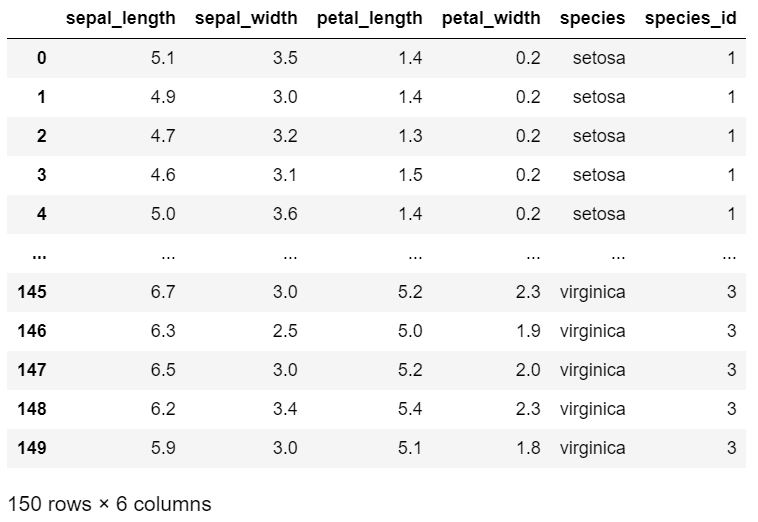Example 1: Plotting default scatter plot on iris dataset.

## Python

 `# importing library``import` `plotly.express as px`` ` `# importing iris dataset from plotly``df ``=` `px.data.iris()`` ` `# plotting the scatter plot on sepal_width ``# and sepal_length and giving color with ``# respect to species``fig ``=` `px.scatter(df, x``=``'sepal_width'``, ``                 ``y``=``'sepal_length'``, ``                 ``color``=``'species'``)`` ` `# showing the plot with default settings``fig.show()`

Output: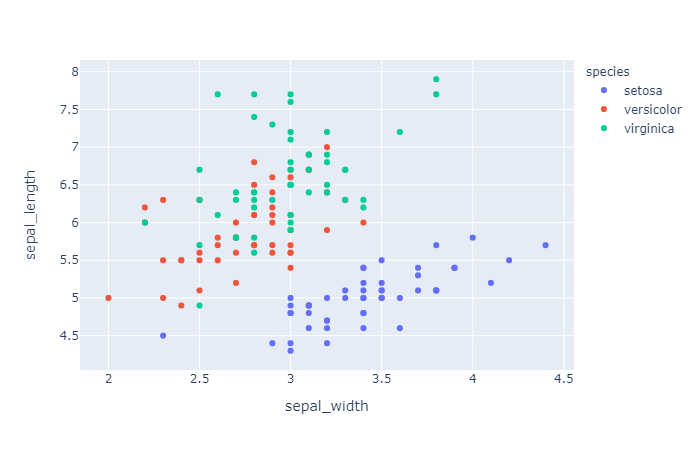Plotting scatter plot on iris dataset and changing marker border color.

## Python

 `# importing library``import` `plotly.express as px`` ` `# importing iris dataset from plotly``df ``=` `px.data.iris()`` ` `# plotting the scatter plot on sepal_width``# and sepal_length and giving color ``# with respect to species``fig ``=` `px.scatter(df, x``=``'sepal_width'``, ``                 ``y``=``'sepal_length'``, ``                 ``color``=``'species'``)`` ` `# setting up marker and in line ``# Attribute giving the width and color of border``fig.update_traces(marker``=``dict``(size``=``10``, ``                              ``line``=``dict``(width``=``3``,``                                        ``color``=``'blue'``)))`` ` `# showing the plot with default settings``fig.show()`

Output: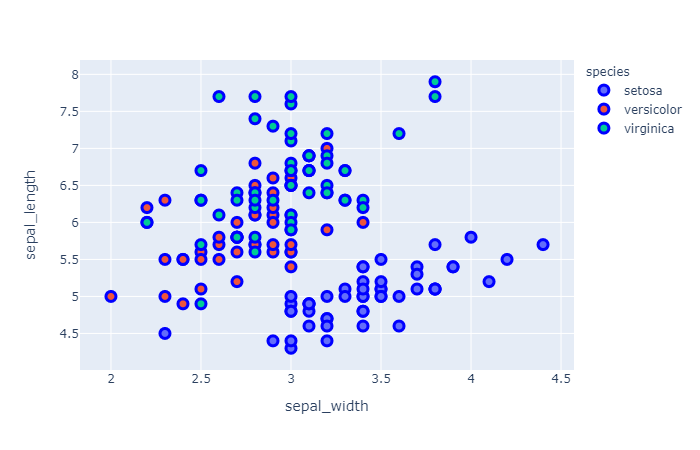In the above code, we had used update_traces() function in that we had given marker attribute for creating the borders in that attribute we had passed the dictionary in which we had passes size=10 for giving the size to the circles, whereas in line we had passed the dictionary in which we had passes width and color, width refers to the width of the border and color refers to the color of the border.

Example 3: Plotting default scatter_3d plot on iris dataset.

## Python

 `# importing library``import` `plotly.express as px`` ` `# importing iris dataset from plotly``df ``=` `px.data.iris()`` ` `# plotting the scatter 3d plot by giving ``# three axis, petal length, petal width ``# and sepal length ``# giving color with respect to species``fig ``=` `px.scatter_3d(df,x``=``'petal_length'``,``                    ``y``=``'petal_width'``,``                    ``z``=``'sepal_length'``,``                    ``color``=``'species'``)`` ` `# showing the plot with default settings``fig.show()`

Output: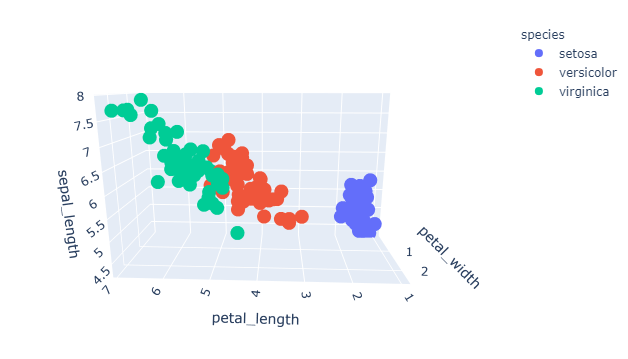Example 4: Plotting scatter_3d plot on iris dataset with marker border color.

## Python

 `# importing library``import` `plotly.express as px`` ` `# importing iris dataset from plotly``df ``=` `px.data.iris()`` ` `# plotting the scatter_3d plot by ``# giving three axis on petal_length, ``# petal_width and sepal_length  ``# and giving color with respect to species``fig ``=` `px.scatter_3d(df,x``=``'petal_length'``,``                    ``y``=``'petal_width'``,``                    ``z``=``'sepal_length'``,``                    ``color``=``'species'``)`` ` `# setting up marker and in line Attribute ``# giving the width and color of border``fig.update_traces(marker``=``dict``(size``=``10``,``                              ``line``=``dict``(width``=``10``,``                                        ``color``=``'red'``)))`` ` `# showing the plot ``fig.show()`

Output: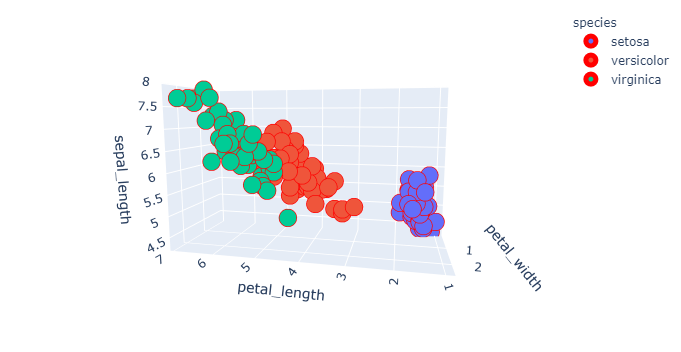My Personal Notes arrow_drop_up